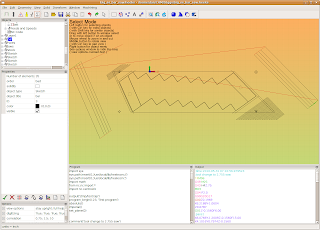## Monday, May 31, 2010

### Roughing and Sawing in the mill

I am making some brass parts on my mill that require some drilling, milling, and sawing with a jeweler's saw. I thought that this would be a good chance to try HeeksCNC out with these operations.
HeeksCNC has some very good operations for drilling and profiling that would be handy for this, but there is nothing in the way of automatic roughing or multi pass sawing. I ended up using some other CAD tricks and HeeksCNC's python scripting to make things work.
Here is the drawing as it imported into HeeksCAD:The stair step shaped part is the thing that I am ultimately cutting out. On the right and left corners of it, I have added (in another CAD program) some roughing passes to cut the corners of my stock off:The roughing actually looks like this on my Bridgeport mill:I also needed to saw some slits in the sides of the part. I wanted to do the sawing with 4 passes of the saw blade on each side. I used my CAD program to offset the final saw path back half the diameter of the saw blade and then offset it 3 additional times by .050".Obviously, it's critical not to retract the saw blade in the Z axes at the wrong time,and I wanted to keep the saw blade down at the right Z level while doing these passes, so I needed to do something to avoid using HeeksCNC's usual profiling method of doing a path, retracting in Z and then doing the next path. I resorted to some Python scripting tricks.
1. I selected the lines that I had drawn in CAD for sawing in HeeksCNC and generated a Profile operation with them. This allowed me to extract the geometric points that I needed.
2. I copied the python script from HeeksCNC and pasted it into my favorite text editor.
3. The script was easy to understand and manipulate- I just wanted a series of 'feed' and 'rapid' in the right spots.I didn't use any loops, because I was lazy....

Here is my sawing script:

comment('tool change to 2.755 saw')
tool_change( id=4)
spindle(300)
feedrate_hv(15, 6)
flush_nc()
clearance = float(0.5)
rapid_down_to_height = float(0.07874015748)
start_depth = float(0)
step_down = float(0.156)
final_depth = float(-0.156)
tool_diameter = float(2.755)

rapid(z=0.1968503937)
rapid(x=6.218827292, y=-1.060352717)
rapid(z=0.07874015748)
feed(z=-0.156)

comment('pass1')
feed(x=6.076573626, y=-1.200936811, z=-0.156)
feed(x=4.101644628, y=0.7974459866, z=-0.156)

comment('Rapid Away')
rapid(x=4.266169148, y=0.9154947677)
rapid(x=6.218827292, y=-1.060352717)

comment('pass2')
feed(x=5.994311367, y=-1.25996122, z=-0.156)
feed(x=4.019382367, y=0.7384215961, z=-0.156)
comment('Rapid Away')
rapid(x=4.266169148, y=0.9154947677)
rapid(x=6.218827292, y=-1.060352717)

comment('pass3')
feed(x=5.912049106, y=-1.31898563, z=-0.156)
feed(x=3.937120107, y=0.6793972059, z=-0.156)

comment('Rapid Away')
rapid(x=4.266169148, y=0.9154947677)
rapid(x=6.218827292, y=-1.060352717)

comment('pass4')
feed(x=5.829786846, y=-1.37801, z=-0.156)
feed(x=3.854857847, y=0.6203728154, z=-0.156)
comment('Rapid Away')
rapid(x=4.266169148, y=0.9154947677)
rapid(z=0.1968503937)

comment('rapid_left')
rapid(z=0.5)
rapid(x=-2.953405945, y=0.07385377598)
rapid(z=0.07874015748)
feed(z=-0.156)

comment('pass1_left')
feed(x=-2.787292165, y=0.1930428677, z=-0.156)
feed(x=-1.668332953, y=-2.357971772, z=-0.156)

comment('rapid away')
rapid(x=-1.834446732, y=-2.477160866)
rapid(x=-2.953405945, y=0.07385377598)

comment('pass2_left')
feed(x=-2.704235276, y=0.2526374134, z=-0.156)
feed(x=-1.585276063, y=-2.298377244, z=-0.156)

comment('rapid away')
rapid(x=-1.834446732, y=-2.477160866)
rapid(x=-2.953405945, y=0.07385377598)

comment('pass3_left')
feed(x=-2.621178386, y=0.3122319591, z=-0.156)
feed(x=-1.502219173, y=-2.238782677, z=-0.156)

comment('rapid away')
rapid(x=-1.834446732, y=-2.477160866)
rapid(x=-2.953405945, y=0.07385377598)

comment('pass4_left')
feed(x=-2.538121535, y=0.3718265047, z=-0.156)
feed(x=-1.419162283, y=-2.17918815, z=-0.156)

comment('rapid away')
rapid(x=-1.834446732, y=-2.477160866)
rapid(x=-2.953405945, y=0.07385377598)
rapid(z=.5)

program_end()

Here is what things looked like on the milling machine: